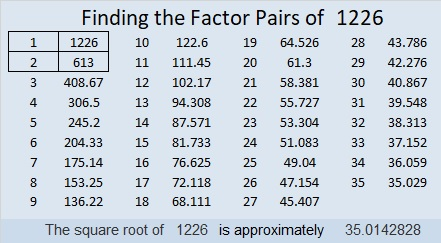# 1226 Happy Birthday to My Sister, Sue

I don’t make puzzles bigger than 12 × 12 very often, but I decided to make this one, a 17 × 17 Mystery Level for my sister’s birthday. I know she can solve smaller ones without any problems, so I wanted to give her a challenge. Happy birthday, Sue. I hope you have a great day and enjoy solving this one.Print the puzzles or type the solution in this excel file: 10-factors-1221-1231

Note that with a bigger table there are several more possible common factors:

Is 4, 8, or 16 the common factor needed for 64 and 32 or for 16 and 48?
Is 7 or 14 the common factor needed for 14 and 70?
Is 6, 10, or 15 the common factor needed for 60 and 90?

As always there is only one solution. The table below will help anyone not familiar with some of the lesser known multiplication facts needed to solve the puzzle.Now I’ll share some information about the number 1226:

• 1226 is a composite number.
• Prime factorization: 1226 = 2 × 613
• The exponents in the prime factorization are 1 and 1. Adding one to each and multiplying we get (1 + 1)(1 + 1) = 2 × 2 = 4. Therefore 1226 has exactly 4 factors.
• Factors of 1226: 1, 2, 613, 1226
• Factor pairs: 1226 = 1 × 1226 or 2 × 613
• 1226 has no square factors that allow its square root to be simplified. √1226 ≈ 35.0142835² + 1² = 1226

1226 is the hypotenuse of a Pythagorean triple:
70-1224-1226 calculated from 2(35)(1), 35² – 1², 35² + 1²